Courses

# Test: Capacitors & Inductors

## 10 Questions MCQ Test Topicwise Question Bank for Electronics Engineering | Test: Capacitors & Inductors

Description
This mock test of Test: Capacitors & Inductors for Electrical Engineering (EE) helps you for every Electrical Engineering (EE) entrance exam. This contains 10 Multiple Choice Questions for Electrical Engineering (EE) Test: Capacitors & Inductors (mcq) to study with solutions a complete question bank. The solved questions answers in this Test: Capacitors & Inductors quiz give you a good mix of easy questions and tough questions. Electrical Engineering (EE) students definitely take this Test: Capacitors & Inductors exercise for a better result in the exam. You can find other Test: Capacitors & Inductors extra questions, long questions & short questions for Electrical Engineering (EE) on EduRev as well by searching above.
QUESTION: 1

### An Inductor works as a ________ circuit for DC supply.

Solution:

Induced voltage across an inductor is zero if the current flowing through it is constant, i.e. Inductor works as a short circuit for DC supply.

QUESTION: 2

### The equivalent circuit of the capacitor shown below is: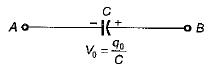Solution:

Due to initial condition, at t = 0 capacitor will act as a constant voltage source (at t = 0, capacitor acts as short-circuit). Hence, option (d) is correct.

QUESTION: 3

### The strength of current in 1 Henry inductor changes at a rate of 1 A/sec. The magnitude of energy stored in the inductor after 3 sec is:

Solution: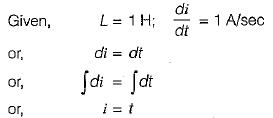∴ Current in the inductor after 3 sec is:

1= 3 A

Hence, energy is stored after 3 sec: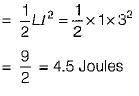QUESTION: 4

The current and voltage profile of an element vs time has been shown in given figure. The element and its value are respectively: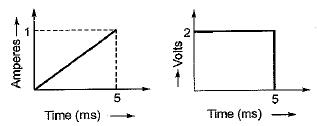Solution:
• Since V is not proportional to R therefore, the element can’t be a resistor.
• At t = 5 ms, even if i ≠ 0, the element behaves as a short circuit therefore, the element can’t be a capacitor (since at t = 0 only capacitor behaves as short circuit).
• The current at t = 0 is zero and at t = 5 ms voltage across the element is zero therefore, the element must be an inductor (at t = 0, an inductor acts as open circuit and at t =∞ it behaves as short circuit).
• From the given voltage and current profile, we have: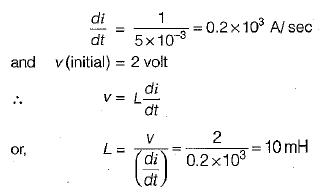QUESTION: 5

Figure shown below exhibits the voltage-time profile of a source to charge a capacitor of 50 μF. The value of charging current in amperes is: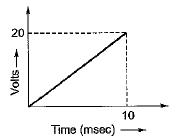Solution:

From given figure: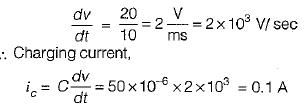QUESTION: 6

The equivalent capacitance across the given terminals A-B is: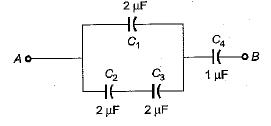Solution:
• The equivalent combination of C2 and C3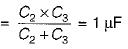• The equivalent combination of this 1 μF and  C1 = 2μF is C1+ 1 μF = 3μF
• Hence, the equivalent capacitance between terminals A and B is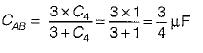QUESTION: 7

The charging time required to charge the equivalent capacitance between the given terminals a-b by a steady direct current of constant magnitude of 10 A is given by: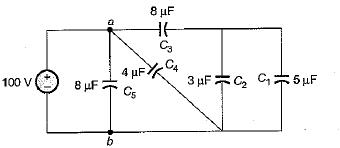Solution:

Equivalent capacitance between terminals a-b is: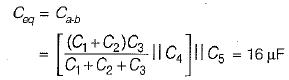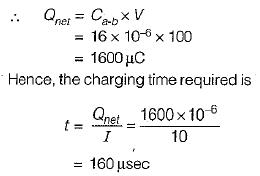QUESTION: 8

An ac voltage of 220 V is applied to a pure inductance of 50 H. If the current is 5 A, the instantaneous value of voltage and current will be respectively given by:

Solution:

Max. value of current: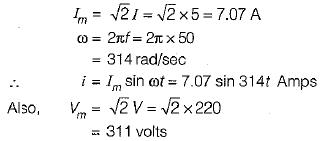Assuming voltage as the reference phasor: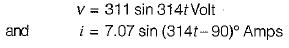QUESTION: 9

The voltage and current through a circuit element is v= 100 sin (314 t + 45°) volts and i = 10 sin (314 t - 45 ° ) amps.
The type of circuit element and its value will be respectively:

Solution: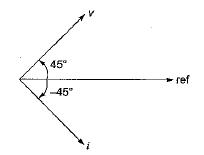The phase difference between v and i is: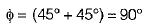Since v leads i therefore, the circuit element is an inductor.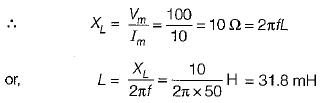QUESTION: 10

The equivalent inductance for the inductive circuit shown below at terminal “ 1 - 2 ” is: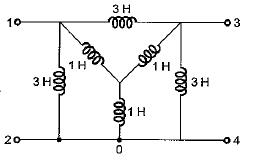Solution:

Converting the internal star connected inductance to an equivalent delta, the circuit reduces as shown below.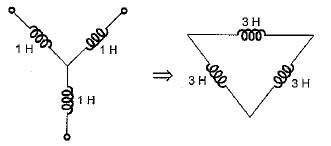Hence, equivalent circuit becomes as shown below.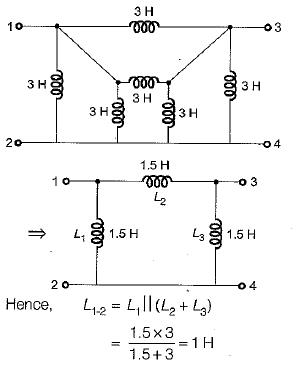• Test